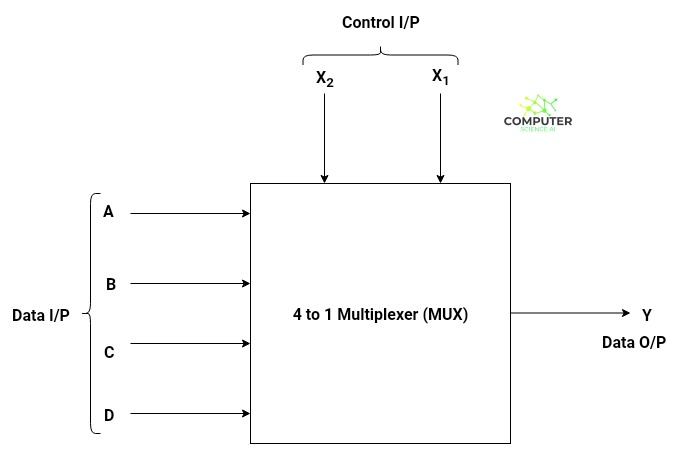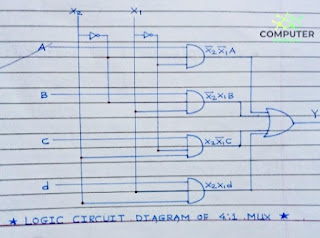# 4 to 1 Multiplexer (MUX) | Truth Table and Block Diagram

4 to 1 Multiplexer is a multiplexer which takes 4 data inputs along with two control inputs and gives only a single data output. Multiplexer is a combinational circuit which take n number of inputs and gives a single output.

In electronics, a multiplexer is a device that selects between several analog or digital input signals and forwards it to a single output line. A multiplexer of n inputs has n select lines, which are used to select which input line to send to the output. It is also called as data selector. It is often abbreviated as MUX.

#### Post Contents

The number of control inputs are determined by the formula 2m = number of data inputs, where m represents the number of control inputs.

Let's find out the number of control inputs :
Here 2m = 4 as there are 4 data inputs and hence it can be easily seen that m=2, so we require 2 control inputs to implement 4 to 1 multiplexer.

## 4 to 1 Multiplexer Block Diagram4 to 1 mux ic number : IC 74352 and IC 74153 are used as 4:1 mux ic.

## 4 to 1 Multiplexer (mux) Using 2 to 1 mux Truth Table

2 to 1 MUX Truth Table
Input Output
Data Input Control Input Data Output
A, B 0 A
A, B 1 B

Truth Table of 4 to 1 Multiplexer
Input Output
Data Input Control Input Data Output
X2 X1
A, B, C, D 0 0 A
A, B, C, D 0 1 B
A, B, C, D 1 0 C
A, B, C, D 1 1 D

Output Equation : Y = X2X1A + X2X1B + X2X1C + X2X1D

Above diagram is of SOP (Sum of Products) type. To implement this via logic ciruit diagram we need to use the combination of AND - OR logic gates.

## Circuit Diagram of 4 to 1 Multiplexer

We can draw the circuit diagram of 4 to 1 multiplexer by using two 2 to 1 multiplexer.## List of ICs which provide multiplexing

The 7400 series has several ICs that contain multiplexer(s):

Multiplexer ICs
IC No. Function Output State
74150 16:1 mux Inverted input
74151 8:1 mux Inverted input
74151A 8:1 mux Complementary Outputs
74352 Dual 4:1 mux Inverted input
74153 Dual 4:1 mux Same as input
74157 Quad 2:1 mux Same as input
74158 Quad 2:1 mux Inverted input

## Applications of Multiplexer

Multiplexers are usually used in communication systems along with the Demultiplexer to carry out the process of data transmission.
1. Communication Systems
2. Computer Memory
3. Telephone Networks
4. Transmission of data from satellites to ground computers using GPS (Global Positioning System).